Next: Protostellar Evolution of Supercritical Up: Dynamical Collapse Previous: Dynamical Collapse   Contents

## Inside-out Collapse Solution

In 1977 Shu found another self-similar solution which is realized after a central protostar with infinitesimal mass is formed in the singular isothermal sphere solution. The gas begins to accrete to the protostar. Outside the region where the accretion occurs, the initial SIS is kept unchanged, since the SIS is a hydrostatic solution. And the front of accretion expands radially outward in time. Since the inflow region expand outwardly, he called it the inside-out collapse solution. In Figure 4.7, the evolution is shown for density and inflow velocity. This solution gives the evolution of a protostellar core formed in a subcritical cloud/cloud core.

Using equations (4.65) and (4.66) and assuming the inflow velocity should reduce at large radius, we obtain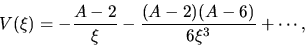(4.69)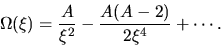(4.70)

Since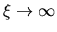means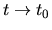(if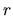is finite),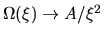means that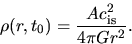(4.71)

Comparing with the SIS, when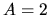this gives the SIS and when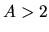this gives a density distribution in which the pressure is inefficient and the cloud is contracting. The solution withis obtained by a procedure as (1) at a sufficiently large radius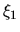, calculate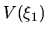and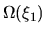. (2) from these values, integrate equations (4.59) and (4.60) inwardly. Figure 4.7 show the solution of this type. The solution withinflow speed is accelerated towards the center. Decreasing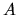(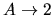), it is shown that an outer part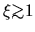reaches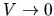. For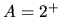, the solution reaches the singular line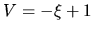at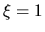(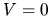) 4.2. Sinceand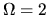at, this solution withconverges to the SIS at. This means that if there is an infinitesimally small amount of excess mass at the center of SIS, the accretion begins from the center while outside a radius the cloud is left static. The inner part of the solution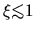,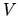and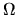are well expressed as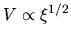and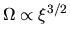.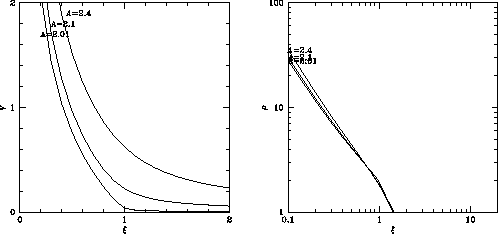The power-law distributions ofandare explained as follows: Conservation of the total energy of an inflowing gas shell is expressed as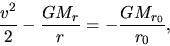(4.72)

where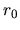denotes the initial radius of a gas shell and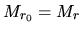represents the mass inside the gas shell. Neglecting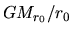compared with the term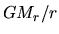, this gives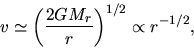(4.73)

where we assumed a major part of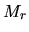comes from the mass of a protostar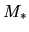, that is,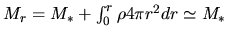Since the average density inside the radius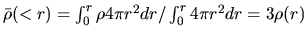for SIS distribution, the time necessary for a gas shell to reach the center is proportional to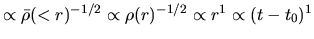, where we used a fact that the front of accretion expands with a constant speed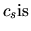. This means that the time necessary for the gas shell to travel from the radius of the accretion wave front to the center is proportional to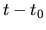. Since the mass of the shell which begins accretion in a unit time is equal to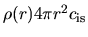and is constant irrespective of. These two facts indicate that the mass accretion rate is constant in time. That is,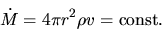(4.74)

Since the mass swept by the sound wave per unit time is equal to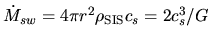, the accretion rate is proportional to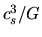. Shu (1977) obtained the accretion rate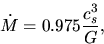(4.75)

for SIS. Using this equation, equations (4.73) and (4.74) indicate that the spatial density distribution is expressed by a power-law as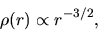(4.76)

which is valid for the region except for the vicinity of the front of the accretion.Next: Protostellar Evolution of Supercritical Up: Dynamical Collapse Previous: Dynamical Collapse   Contents
Kohji Tomisaka 2007-07-08